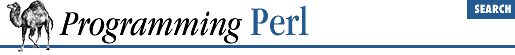home | O'Reilly's CD bookshelfs | FreeBSD | Linux | Cisco | Cisco Exam

#### 3.2.159 stat

```stat ```

FILEHANDLE
```

stat ```

EXPR
```
```

This function returns a 13-element list giving the statistics for a file, either the file opened via ``` FILEHANDLE ``` , or named by ``` EXPR ``` . It's typically used as follows:

```(\$dev,\$ino,\$mode,\$nlink,\$uid,\$gid,\$rdev,\$size,
\$atime,\$mtime,\$ctime,\$blksize,\$blocks)
= stat \$filename;```

Not all fields are supported on all filesystem types. Here are the meanings of the fields:

Field Meaning
``` dev``` Device number of filesystem
``` ino``` Inode number
``` mode``` File mode (type and permissions)
``` nlink``` Number of (hard) links to the file
``` uid``` Numeric user ID of file's owner
``` gid``` Numeric group ID of file's owner
``` rdev``` The device identifier (special files only)
``` size``` Total size of file, in bytes
``` atime``` Last access time since the epoch
``` mtime``` Last modify time since the epoch
``` ctime``` Inode change time (NOT creation time!) since the epoch
``` blksize``` Preferred blocksize for file system I/O
``` blocks``` Actual number of blocks allocated

``` \$dev``` and ``` \$ino``` , taken together, uniquely identify a file. The ``` \$blksize``` and ``` \$blocks``` are likely defined only on BSD-derived filesystems. The ``` \$blocks``` field (if defined) is reported in 512-byte blocks. Note that ``` \$blocks*512``` can differ greatly from ``` \$size``` for files containing unallocated blocks, or "holes", which aren't counted in ``` \$blocks``` .

If stat is passed the special filehandle consisting of an underline, no actual stat (2) is done, but the current contents of the stat structure from the last stat or stat -based file test (the ``` -x``` operators) are returned.

The following example first stats ``` \$file``` to see whether it is executable. If it is, it then pulls the device number out of the existing stat structure and tests it to see whether it looks like a Network File System (NFS). Such filesystems tend to have negative device numbers.

```if (-x \$file and (\$d) = stat(_) and \$d < 0) {
print "\$file is executable NFS file\n";
}```

Hint: if you need only the size of the file, check out the ``` -s``` file test operator, which returns the size in bytes directly. There are also file tests that return the ages of files in days.